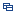| Recent Visit History | Join Audited Suppliers | Help | Region Guide
» » » New Products

181.

Hr Coating[Nov 25, 2021]

Plano-convex is close to best form for a focusing lens. Focusing or optical imaging Focal Length: F = R/(n-1) N: Refractive index R: Radius curvature Plano-convex lenses are useful for a wide range of ...

182.

Aspheric Supplier[Nov 25, 2021]

Plano-convex is close to best form for a focusing lens. Focusing or optical imaging Focal Length: F = R/(n-1) N: Refractive index R: Radius curvature Plano-convex lenses are useful for a wide range of ...

183.

Optical Coating[Nov 25, 2021]

Plano-convex is close to best form for a focusing lens. Focusing or optical imaging Focal Length: F = R/(n-1) N: Refractive index R: Radius curvature Plano-convex lenses are useful for a wide range of ...

184.

Narrow Band Filters[Nov 25, 2021]

Plano-convex is close to best form for a focusing lens. Focusing or optical imaging Focal Length: F = R/(n-1) N: Refractive index R: Radius curvature Plano-convex lenses are useful for a wide range of ...

185.

Beam Splitter[Nov 25, 2021]

Plano-convex is close to best form for a focusing lens. Focusing or optical imaging Focal Length: F = R/(n-1) N: Refractive index R: Radius curvature Plano-convex lenses are useful for a wide range of ...

186.

Customization Cth YAG Laser Rod Chromium Thulium Homium-Doped YAG[Dec 16, 2021]

Description of CTH YAG rod VY Optics can make kinds of YAG crystals as per your request, such as CTH:YAG, Nd:YAG, Er:YAG and so on. CTH:YAG-Chromium, Thulium, Holmium: Yttrium Aluminum Garnet, it is a new crystal ...

Company: VY Optoelectronics Co., Ltd.

187.

Negative Meniscus Cylindrical[Dec 16, 2021]

Plano-convex is close to best form for a focusing lens. Focusing or optical imaging Focal Length: F = R/(n-1) N: Refractive index R: Radius curvature Plano-convex lenses are useful for a wide range of ...

188.

Plano-Concave Cylindrical Lenses[Dec 16, 2021]

Plano-convex is close to best form for a focusing lens. Focusing or optical imaging Focal Length: F = R/(n-1) N: Refractive index R: Radius curvature Plano-convex lenses are useful for a wide range of ...

189.

Hemisphere Lenses[Dec 16, 2021]

Plano-convex is close to best form for a focusing lens. Focusing or optical imaging Focal Length: F = R/(n-1) N: Refractive index R: Radius curvature Plano-convex lenses are useful for a wide range of ...

190.

Ball Lenses[Dec 16, 2021]

Plano-convex is close to best form for a focusing lens. Focusing or optical imaging Focal Length: F = R/(n-1) N: Refractive index R: Radius curvature Plano-convex lenses are useful for a wide range of ...

191.

Plano-Convex Cylindrical Lenses[Dec 16, 2021]

Plano-convex is close to best form for a focusing lens. Focusing or optical imaging Focal Length: F = R/(n-1) N: Refractive index R: Radius curvature Plano-convex lenses are useful for a wide range of ...

192.

Bi-Convex Cylindrical Lenses[Dec 16, 2021]

Plano-convex is close to best form for a focusing lens. Focusing or optical imaging Focal Length: F = R/(n-1) N: Refractive index R: Radius curvature Plano-convex lenses are useful for a wide range of ...

193.

Cylindrical Lens Manufacture[Nov 24, 2021]

Plano-convex is close to best form for a focusing lens. Focusing or optical imaging Focal Length: F = R/(n-1) N: Refractive index R: Radius curvature Plano-convex lenses are useful for a wide range of ...

194.

Cylindrical Supplier for Sale[Nov 24, 2021]

Plano-convex is close to best form for a focusing lens. Focusing or optical imaging Focal Length: F = R/(n-1) N: Refractive index R: Radius curvature Plano-convex lenses are useful for a wide range of ...

195.

Optical Window[Nov 25, 2021]

Plano-convex is close to best form for a focusing lens. Focusing or optical imaging Focal Length: F = R/(n-1) N: Refractive index R: Radius curvature Plano-convex lenses are useful for a wide range of ...

196.

Window Manufacturer[Nov 25, 2021]

Plano-convex is close to best form for a focusing lens. Focusing or optical imaging Focal Length: F = R/(n-1) N: Refractive index R: Radius curvature Plano-convex lenses are useful for a wide range of ...

197.

Dome Lens[Nov 25, 2021]

Plano-convex is close to best form for a focusing lens. Focusing or optical imaging Focal Length: F = R/(n-1) N: Refractive index R: Radius curvature Plano-convex lenses are useful for a wide range of ...

198.

Aspheric Lens Manufacture[Nov 25, 2021]

Plano-convex is close to best form for a focusing lens. Focusing or optical imaging Focal Length: F = R/(n-1) N: Refractive index R: Radius curvature Plano-convex lenses are useful for a wide range of ...

199.

Window Supplier[Nov 25, 2021]

Plano-convex is close to best form for a focusing lens. Focusing or optical imaging Focal Length: F = R/(n-1) N: Refractive index R: Radius curvature Plano-convex lenses are useful for a wide range of ...

200.

Optical Glass Cylindrical Lens[Dec 07, 2021]

Plano-convex is close to best form for a focusing lens. Focusing or optical imaging Focal Length: F = R/(n-1) N: Refractive index R: Radius curvature Plano-convex lenses are useful for a wide range of ...

Hot Region Index: ABCDEFGHIJKLMNPQRSTUWXYZ